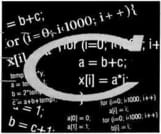0
Thanks

A few words of thanks would be greatly appreciated.

# C Language - Generate random values## Issue

I want to generate a number between 0 and 4 (possible values: 0,1,2,3,4) that will be then stored in the "nbr" variable.
• How to achieve the following using the rand () function?

## Solution

Try this:
```#include <stdio.h>
#include <stdlib.h>
#include <time.h>
#include <conio.h>

int Random (int _iMin, int _iMax)
{
return (_iMin + (rand () % (_iMax-_iMin+1)));
}

int main (void)
{
int iRandom;

srand (time (NULL));
iRandom = Random (50,100);
printf ("Random : %d", iRandom);
getch();
return 0;
} ```

Thanks to scoubidou944 for this tip.
0
Thanks

A few words of thanks would be greatly appreciated.